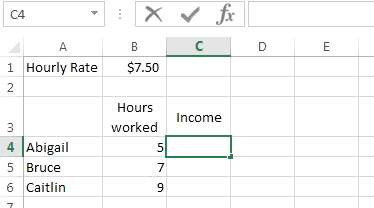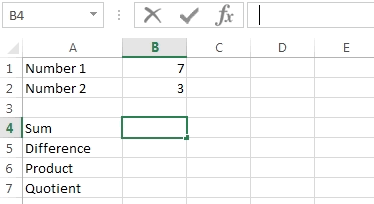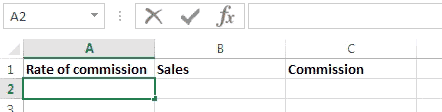### Excel formula

#### Absolute Reference | # gomaths.net/4241

An absolute reference in a formula does not change when the formula is copied or filled.

An absolute reference uses `\$` signs,

`=\$B\$1` the column and row do not change.
`=\$B1` the column does not change
`=B\$1` the row does not change#### Basic Operations | # gomaths.net/4243

Addition: `=B1+B2`

Subtraction: `=B1-B2`

Multiplication: `=B1*B2`

Division: `=B1/B2`#### Commission | # gomaths.net/4221

Calculate the commission on sales.

`=A2*B2`

where `A2` is the rate of commission (entered as a percentage)
`B2` is the amount of sales